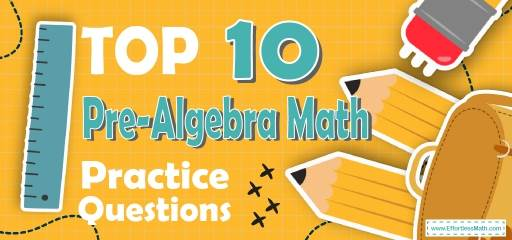# Top 10 Pre-Algebra Practice QuestionsPreparing for Pre-Algebra test? The best way to prepare for your Pre-Algebra test is to work through as many Pre-Algebra practice questions as possible. Here are the top 10 Pre-Algebra practice questions to help you review the most important Pre-Algebra concepts. These Pre-Algebra practice questions are designed to cover mathematics concepts and topics that are found on the actual test. The questions have been fully updated to reflect the latest 2022 Pre-Algebra guidelines. Answers and full explanations are provided at the end of the post.

Start your Pre-Algebra test prep journey right now with these sample Pre-Algebra questions.

The Absolute Best Book to Ace the Pre-Algebra

## Pre-Algebra Practice Questions

1- What is the value of the expression $$(5(x-2y)+(2-x)^2)$$ when $$x=3$$ and $$y=-2$$ ?

A. 20

B. 50

C. 1156

D. 36

2- What is the slope of a line that is perpendicular to the line
$$4x-2y=12$$ ?

A. -2

B. 4

C. 2

D.$$-\frac{1}{2}$$

3- Which of the following is equal to the expression below?
$$(2x+2y)(2x-y)$$

A. $$4x^2- 2y^2$$

B. $$2x^2+6xy-2y^2$$

C. $$24x^2+2xy-2y^2$$

D. $$4x^2+2xy-2y^2$$

4- What is the product of all possible values of x in the following equation?
$$|x-10| = 3$$

A. 3

B. 7

C. 13

D. 91

5- $$[6 \times (- 24) + 8] – (-4) + [4 \times 5] \div 2 = ?$$

A. 111

B. $$-112$$

C. $$-122$$

D. $$-144$$

6- What is the value of x in the following system of equations?
$$2x+5y=11$$
$$4x-2y=-14$$

A. $$-1$$

B. 1

C. 4

D. $$-2$$

7- What is the median of these numbers? 2, 27, 28, 19, 67, 44, 35

A. 19

B. 28

C. 44

D. 35

8- A swimming pool holds 2,000 cubic feet of water. The swimming pool is 25 feet long and 10 feet wide. How deep is the swimming pool?_______

9- The area of a circle is $$64 π$$. Which of the following is the circumference of the circle?

A. 8$$\pi$$

B. 12$$\pi$$

C. 16$$\pi$$

D. 64$$\pi$$

10- What is the value of $$3^6$$ ?______

Best Algebra Prep Resource

1- D
Plug in the value of $$x$$ and $$y$$.
$$x=3$$ and $$y=-2$$
$$(5(x-2y)+(2-x)^2)=(5(3-2(-2))+(2-3)^2)=(5(3+4)+(-1)^2) = 35+1=36$$

2- D
The equation of a line in slope intercept form is: $$y=mx+b$$
Solve for $$y$$.
$$4x-2y=12 {\Rightarrow} -2y=12-4x {\Rightarrow} y=(12-4x){\div}(-2) {\Rightarrow} y=2x-6$$
The slope of this line is 2.
The product of the slopes of two perpendicular lines is -1.
Therefore, the slope of a line that is perpendicular to this line is:
$$m_{1} {\times} m_{2} = -1 {\Rightarrow} 2 {\times} m_{2} = -1 {\Rightarrow} m_{2} = \frac{-1}{2}$$

3- D
Use FOIL method.
$$(2x+2y)(2x-y) = 4x^2-2xy+4xy-2y^2=4x^2+2xy-2y^2$$

4- D
To solve absolute values equations, write two equations.
$$x-10$$ could be positive 3, or negative 3. Therefore,
$$x-10=3 \Rightarrow x=13$$
$$x-10=-3 \Rightarrow x=7$$
Find the product of solutions: $$7 \times 13 = 91$$

5- C
Use PEMDAS (order of operation):
$$[6 {\times} (- 24) + 8] – (- 4) + [4 × 5] {\div} 2$$
$$[- 144 + 8] – (- 4) +  {\div} 2 = [- 144 + 8] – (- 4) + 10$$
$$[- 136] – (- 4) + 10 = [- 136] + 4 + 10 = – 122$$

6- D
Solving Systems of Equations by Elimination
Multiply the first equation by (-2), then add it to the second equation.
$${-2(2x+5y= 11) \ 4x-2y=-14} {\Rightarrow} {-4x-10y= -22 \ 4x-2y=-14} {\Rightarrow} {-12y= -36} {\Rightarrow} {y= 3}$$
Plug in the value of y into one of the equations and solve for x.
$$2x+5(3)= 11 {\Rightarrow} 2x+15= 11 {\Rightarrow} 2x= -4 {\Rightarrow} x= -2$$

7- B
Write the numbers in order:
2, 19, 27, 28, 35, 44, 67
Median is the number in the middle. So, the median is 28

8- 8
Use formula of rectangle prism volume.
$$V = (length) (width) (height) {\Rightarrow} 2000 = (25) (10) (height) {\Rightarrow} height = 2000 {\div} 250 = 8$$

9- C
The area of the circle is $$16 π$$. Use the formula of areas of circles.
$$Area = πr^2 ⇒ 64 π> πr^2⇒ 64 > r^2⇒ r < 8$$
The radius of the circle is 8. Let’s put 8 for the radius. Now, use the circumference formula:
$$Circumference =2πr=2π (8)=16π$$

10- 729
$$(3^6 ) = 3 × 3 × 3 × 3 × 3 × 3 = 729$$

Looking for the best resource to help you succeed on the Pre-Algebra Math test?

The Best Books to Ace Algebra

### What people say about "Top 10 Pre-Algebra Practice Questions - Effortless Math: We Help Students Learn to LOVE Mathematics"?

No one replied yet.

X
49% OFF

Limited time only!

Save Over 49%

SAVE $34 It was$69.99 now it is \$35.99